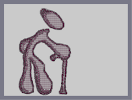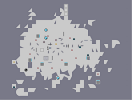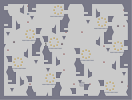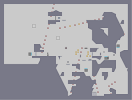### Old timers.Hover over the thumbnail for a full-size version.

Author BE_nSPIRED author:be_nspired grampa inspired n-art ninja old rated 2011-06-24 2011-06-24 4 by 26 people. \$Old timers.#BE_nSPIRED#ninja#00000000000000000000000000000000000000000000000000000000000000000000000000000000000000000000000000000000000000000000000000000000000000000000000000000000000000000000000000000000000000000000000000000000000000000000000000000000000000000000000000000000000000000000000000000000000000000000000000000000000000000000000000000000000000000000000000000000000000000000000000000000000000000000000000000000000000000000000000000000000000000000000000000000000000000000000000000000000000000000000000000000000000000000000000000000000000000000000000000000000000000000000000000000000000000000000000000000000000000000000000000000000000000000000000000000000000000000000000000000000000000000000000000000000000000000000000000000000000000|10^353,174!10^259,63!10^248,64!10^245,67!10^245,74!10^245,79!10^248,85!10^250,90!10^254,95!10^256,97!10^258,104!10^262,107!10^264,115!10^266,117!10^268,122!10^272,126!10^272,126!10^274,130!10^276,133!10^281,137!10^283,139!10^285,143!10^287,145!10^291,149!10^293,152!10^295,154!10^299,157!10^300,159!10^305,162!10^308,164!10^316,165!10^317,165!10^318,166!10^322,168!10^326,169!10^329,171!10^333,171!10^337,173!10^343,173!10^347,174!10^352,174!10^356,174!10^363,174!10^365,174!10^365,172!10^367,170!10^372,168!10^377,162!10^379,161!10^381,156!10^381,152!10^381,148!10^381,145!10^381,140!10^379,137!10^377,132!10^374,131!10^370,122!10^367,119!10^367,116!10^363,111!10^361,108!10^358,105!10^356,101!10^352,95!10^350,95!10^344,89!10^341,87!10^339,85!10^335,81!10^333,81!10^333,79!10^330,79!10^253,55!10^253,55!10^250,68!10^247,64!10^323,76!10^323,74!10^316,71!10^311,69!10^304,67!10^295,65!10^290,65!10^285,61!10^275,57!10^270,57!10^266,55!10^262,55!10^258,56!10^259,68!10^254,83!10^257,96!10^257,101!10^261,99!10^259,95!10^259,88!10^260,81!10^262,78!10^264,74!10^268,74!10^275,70!10^279,70!10^284,70!10^291,70!10^282,70!10^275,68!10^260,71!10^253,79!10^253,75!10^266,67!10^270,64!10^279,66!10^303,69!10^299,69!10^287,67!10^273,63!10^267,60!10^282,177!10^279,177!10^273,178!10^263,179!10^258,179!10^259,180!10^252,183!10^247,183!10^244,184!10^234,187!10^234,188!10^237,188!10^237,188!10^234,188!10^232,190!10^232,191!10^229,192!10^227,195!10^225,196!10^225,196!10^223,199!10^220,200!10^219,202!10^219,205!10^215,208!10^213,210!10^211,212!10^206,215!10^204,217!10^204,218!10^198,221!10^195,222!10^192,223!10^188,224!10^183,228!10^180,228!10^177,230!10^172,231!10^170,232!10^169,232!10^166,234!10^160,236!10^159,236!10^155,236!10^151,236!10^147,238!10^146,239!10^144,240!10^141,243!10^138,245!10^134,246!10^130,248!10^128,249!10^126,254!10^126,256!10^126,256!10^124,260!10^122,265!10^120,269!10^120,272!10^120,275!10^120,279!10^120,282!10^120,287!10^120,289!10^120,291!10^120,294!10^121,303!10^123,305!10^125,307!10^129,312!10^130,312!10^135,316!10^137,317!10^199,226!10^194,230!10^190,236!10^187,241!10^185,243!10^181,247!10^179,249!10^176,253!10^176,256!10^176,258!10^172,265!10^172,271!10^165,274!10^165,278!10^165,279!10^163,285!10^161,289!10^157,294!10^153,301!10^151,304!10^149,309!10^149,312!10^149,304!10^154,295!10^158,290!10^161,285!10^165,277!10^166,274!10^170,266!10^175,256!10^178,250!10^188,238!10^130,465!10^131,466!10^133,462!10^135,458!10^135,453!10^135,453!10^137,453!10^139,448!10^139,445!10^139,441!10^140,437!10^143,432!10^143,428!10^143,424!10^143,420!10^143,416!10^143,415!10^145,411!10^145,409!10^145,401!10^145,401!10^145,399!10^145,389!10^143,387!10^143,387!10^143,378!10^144,375!10^144,369!10^144,362!10^144,362!10^144,361!10^142,353!10^142,353!10^142,351!10^141,347!10^141,342!10^143,334!10^144,330!10^145,323!10^149,314!10^152,309!10^147,314!10^147,322!10^141,335!10^138,317!10^129,307!10^129,307!10^125,304!10^124,302!10^124,294!10^124,293!10^126,301!10^130,305!10^125,275!10^123,275!10^123,268!10^128,262!10^128,262!10^132,260!10^137,255!10^143,249!10^143,249!10^148,246!10^152,244!10^164,239!10^176,239!10^184,235!10^179,238!10^164,238!10^155,244!10^125,279!10^131,470!10^127,472!10^127,473!10^124,476!10^124,480!10^124,483!10^120,488!10^120,495!10^120,496!10^120,498!10^118,501!10^118,506!10^118,510!10^118,511!10^118,515!10^118,520!10^119,523!10^120,526!10^121,529!10^122,533!10^123,535!10^124,537!10^127,542!10^128,544!10^132,547!10^135,551!10^135,552!10^138,553!10^140,553!10^144,554!10^146,554!10^143,550!10^139,543!10^136,539!10^131,536!10^131,533!10^131,532!10^129,528!10^127,523!10^126,520!10^124,513!10^122,509!10^122,505!10^124,495!10^126,490!10^132,475!10^133,471!10^144,343!10^144,343!10^146,340!10^150,335!10^152,327!10^154,317!10^156,309!10^157,302!10^160,294!10^163,284!10^164,285!10^153,326!10^151,341!10^151,346!10^149,359!10^147,368!10^147,370!10^147,384!10^145,399!10^146,407!10^144,407!10^144,407!10^148,401!10^150,394!10^150,390!10^150,384!10^150,380!10^148,374!10^146,372!10^146,367!10^146,362!10^147,357!10^148,351!10^150,341!10^152,335!10^152,327!10^153,319!10^154,314!10^156,309!10^157,306!10^158,302!10^160,298!10^144,553!10^148,556!10^149,557!10^156,558!10^156,558!10^158,558!10^159,558!10^161,559!10^163,559!10^165,559!10^165,559!10^169,559!10^170,559!10^172,559!10^177,559!10^184,555!10^194,551!10^196,551!10^189,552!10^183,556!10^184,556!10^198,550!10^198,547!10^198,544!10^198,543!10^198,539!10^198,535!10^198,534!10^199,526!10^199,519!10^199,518!10^199,515!10^196,512!10^196,510!10^196,509!10^196,506!10^194,501!10^192,499!10^192,495!10^192,493!10^192,489!10^192,487!10^188,485!10^188,483!10^188,478!10^186,478!10^188,481!10^176,350!10^177,351!10^177,353!10^180,356!10^181,358!10^181,365!10^181,367!10^181,370!10^181,376!10^181,377!10^183,382!10^183,387!10^183,391!10^184,395!10^185,397!10^185,401!10^185,402!10^185,403!10^185,408!10^185,413!10^183,416!10^183,420!10^183,425!10^183,430!10^183,433!10^183,440!10^183,444!10^183,449!10^183,451!10^183,453!10^184,457!10^184,464!10^188,466!10^188,470!10^188,470!10^186,472!10^186,472!10^184,464!10^184,460!10^184,453!10^184,449!10^286,177!10^287,177!10^291,177!10^291,177!10^291,182!10^293,186!10^293,188!10^289,193!10^287,195!10^283,200!10^283,203!10^283,206!10^283,213!10^283,218!10^288,224!10^290,227!10^294,231!10^298,235!10^300,237!10^304,241!10^308,244!10^312,246!10^314,248!10^316,251!10^319,251!10^322,254!10^326,257!10^329,259!10^330,259!10^334,262!10^340,263!10^340,263!10^342,265!10^344,265!10^348,265!10^350,265!10^355,265!10^356,265!10^360,265!10^367,266!10^373,268!10^377,269!10^383,271!10^392,272!10^399,274!10^403,276!10^405,278!10^410,283!10^413,287!10^417,293!10^417,294!10^417,300!10^417,304!10^419,309!10^420,313!10^420,315!10^421,319!10^417,324!10^414,328!10^413,331!10^413,332!10^413,333!10^184,357!10^185,359!10^185,360!10^188,365!10^189,365!10^190,367!10^195,369!10^195,371!10^195,374!10^196,377!10^197,378!10^199,380!10^203,385!10^203,385!10^203,389!10^205,394!10^207,397!10^207,400!10^207,404!10^207,408!10^207,413!10^207,417!10^209,420!10^210,422!10^210,424!10^209,434!10^209,434!10^209,431!10^209,431!10^215,436!10^213,436!10^211,439!10^211,440!10^211,441!10^211,444!10^211,452!10^211,454!10^211,455!10^211,457!10^211,458!10^211,466!10^211,468!10^213,471!10^215,472!10^215,473!10^215,476!10^215,477!10^215,484!10^215,485!10^216,487!10^216,490!10^217,493!10^218,493!10^219,496!10^219,499!10^219,499!10^219,499!10^219,506!10^219,506!10^221,510!10^221,512!10^221,515!10^222,517!10^222,519!10^222,519!10^223,523!10^225,526!10^225,530!10^226,531!10^227,535!10^227,540!10^230,543!10^231,544!10^232,546!10^233,548!10^237,552!10^238,553!10^240,555!10^243,557!10^246,559!10^249,560!10^252,562!10^260,565!10^262,565!10^262,565!10^267,565!10^274,565!10^275,565!10^278,562!10^302,552!10^304,546!10^306,543!10^287,560!10^287,560!10^292,558!10^295,556!10^299,552!10^300,552!10^291,557!10^291,558!10^289,559!10^283,562!10^283,563!10^264,563!10^252,561!10^247,558!10^241,555!10^237,550!10^236,550!10^236,545!10^234,540!10^230,535!10^230,531!10^230,529!10^228,517!10^228,515!10^226,511!10^226,504!10^226,503!10^224,500!10^224,493!10^222,488!10^222,483!10^220,478!10^220,472!10^220,467!10^220,466!10^220,460!10^221,454!10^221,443!10^222,436!10^220,433!10^217,425!10^213,416!10^213,411!10^213,408!10^210,404!10^208,401!10^231,525!10^234,529!10^238,536!10^239,542!10^241,543!10^250,551!10^256,553!10^261,554!10^269,554!10^279,554!10^287,552!10^287,553!10^283,555!10^272,557!10^263,555!10^253,549!10^248,547!10^241,541!10^241,537!10^236,532!10^234,526!10^234,519!10^235,525!10^241,535!10^256,554!10^231,518!10^301,545!10^301,545!10^300,545!10^289,551!10^284,555!10^277,558!10^269,560!10^304,544!10^304,542!10^304,539!10^305,536!10^309,532!10^310,529!10^310,526!10^310,523!10^310,518!10^311,515!10^311,510!10^311,503!10^311,499!10^311,495!10^309,492!10^307,489!10^303,485!10^303,482!10^300,477!10^297,474!10^294,470!10^291,468!10^288,457!10^285,454!10^285,455!10^286,460!10^288,465!10^264,422!10^266,424!10^266,427!10^268,431!10^270,435!10^275,438!10^278,443!10^282,449!10^287,458!10^287,458!10^283,453!10^278,445!10^273,435!10^268,429!10^266,421!10^265,414!10^265,411!10^265,409!10^265,402!10^265,395!10^265,388!10^258,383!10^255,380!10^252,377!10^207,331!10^207,336!10^214,339!10^216,342!10^218,345!10^224,348!10^228,351!10^229,353!10^233,358!10^236,359!10^240,363!10^244,365!10^248,370!10^252,373!10^257,379!10^254,379!10^246,370!10^237,364!10^221,348!10^209,339!10^202,332!10^202,332!10^202,330!10^200,328!10^200,324!10^200,319!10^197,314!10^197,310!10^197,307!10^197,302!10^197,301!10^197,300!10^200,294!10^200,293!10^201,290!10^203,287!10^206,282!10^206,282!10^207,279!10^209,274!10^211,270!10^212,269!10^216,264!10^218,262!10^220,260!10^224,257!10^226,257!10^232,255!10^234,253!10^238,248!10^239,248!10^247,248!10^252,248!10^261,248!10^263,249!10^266,252!10^269,254!10^275,260!10^277,261!10^273,252!10^265,252!10^257,252!10^240,251!10^275,261!10^283,261!10^283,263!10^284,267!10^286,268!10^287,269!10^290,273!10^290,274!10^294,276!10^294,277!10^298,278!10^300,280!10^304,282!10^306,285!10^308,289!10^310,290!10^312,291!10^314,295!10^316,296!10^318,298!10^318,298!10^322,302!10^323,304!10^326,308!10^326,308!10^328,309!10^330,316!10^330,317!10^331,320!10^333,321!10^336,323!10^338,324!10^344,328!10^345,330!10^348,332!10^352,334!10^354,336!10^357,339!10^360,341!10^363,343!10^368,348!10^370,351!10^373,353!10^377,354!10^385,355!10^390,356!10^391,356!10^401,356!10^402,354!10^407,347!10^411,344!10^415,337!10^416,332!10^416,334!10^413,339!10^410,344!10^405,348!10^403,348!10^395,353!10^388,353!10^378,351!10^368,347!10^358,340!10^352,333!10^359,560!10^363,560!10^365,560!10^371,560!10^374,561!10^381,561!10^383,561!10^390,561!10^393,562!10^396,562!10^398,560!10^398,558!10^398,556!10^396,548!10^393,546!10^392,542!10^389,536!10^389,533!10^389,530!10^389,526!10^389,535!10^390,543!10^390,551!10^380,549!10^375,548!10^367,552!10^361,554!10^358,552!10^358,550!10^361,547!10^364,542!10^366,537!10^367,536!10^367,532!10^367,529!10^370,523!10^370,521!10^372,517!10^372,514!10^372,503!10^372,499!10^372,497!10^374,507!10^374,502!10^374,497!10^374,495!10^374,486!10^374,483!10^374,477!10^374,472!10^374,462!10^374,461!10^376,450!10^376,448!10^378,451!10^374,461!10^374,456!10^374,450!10^376,445!10^378,438!10^378,437!10^378,432!10^378,429!10^378,420!10^378,418!10^378,417!10^376,410!10^376,410!10^377,403!10^377,402!10^377,393!10^378,389!10^379,383!10^379,377!10^381,371!10^381,367!10^381,361!10^378,360!10^370,356!10^358,345!10^355,346!10^348,344!10^343,344!10^337,347!10^330,351!10^330,353!10^326,358!10^324,362!10^322,363!10^322,363!10^325,354!10^329,349!10^336,347!10^321,362!10^318,362!10^314,362!10^310,358!10^307,355!10^307,351!10^304,349!10^304,344!10^304,342!10^305,339!10^309,335!10^312,334!10^315,332!10^317,330!10^322,330!10^329,328!10^331,328!10^331,328!10^326,328!10^316,334!10^309,339!10^321,336!10^314,341!10^309,346!10^391,524!10^391,524!10^389,522!10^389,518!10^389,515!10^389,513!10^389,507!10^389,501!10^389,495!10^389,493!10^389,488!10^389,485!10^390,477!10^390,474!10^390,468!10^392,461!10^393,457!10^393,452!10^393,449!10^393,442!10^393,442!10^393,440!10^393,438!10^394,436!10^395,433!10^395,431!10^395,430!10^393,430!10^393,424!10^393,419!10^394,417!10^395,415!10^395,409!10^395,409!10^396,401!10^396,401!10^398,393!10^398,393!10^398,363!10^398,367!10^400,371!10^400,375!10^398,381!10^398,386!10^398,392!10^396,398!10^394,407!10^394,415!10^394,421!10^394,435!10^394,439!10^375,547!10^375,544!10^375,537!10^375,532!10^375,527!10^375,526!10^375,466!3^315,76!3^311,76!3^304,76!3^299,77!3^294,79!3^287,79!3^279,81!3^278,82!3^276,84!3^273,87!3^269,92!3^267,103!3^267,106!3^267,113!3^267,98!3^267,86!3^273,75!3^285,75!3^172,243!3^172,243!3^170,245!3^164,249!3^162,251!3^156,252!3^153,254!3^149,257!3^143,263!3^141,264!3^139,271!3^136,274!3^136,283!3^136,291!3^136,294!3^141,302!3^144,307!3^144,307!3^138,302!3^131,288!3^171,552!3^166,547!3^160,547!3^159,547!3^157,545!3^153,543!3^150,539!3^146,536!3^146,533!3^143,533!3^143,528!3^140,525!3^137,521!3^134,512!3^134,509!3^134,506!3^134,503!3^134,499!3^134,496!3^134,496!3^135,492!3^137,488!3^138,486!3^141,482!3^141,480!3^142,480!3^143,467!3^143,467!3^145,467!3^147,440!3^147,465!3^147,448!3^147,442!3^147,453!3^147,461!3^150,436!3^154,424!3^154,418!3^156,408!3^159,392!3^159,384!3^159,367!3^159,385!3^156,397!3^156,415!3^161,393!3^159,373!3^160,389!3^142,478!3^142,474!3^154,432!3^285,189!3^280,189!3^274,189!3^267,190!3^259,193!3^253,198!3^251,198!3^250,198!3^245,198!3^240,202!3^234,204!3^231,206!3^225,209!3^222,216!3^220,216!3^218,218!3^214,220!3^212,223!3^212,227!3^208,233!3^206,237!3^202,240!3^197,247!3^195,248!3^190,255!3^190,255!3^187,261!3^187,263!3^184,265!3^178,271!3^170,279!3^176,273!3^176,277!3^176,286!3^169,289!3^167,292!3^167,294!3^156,361!3^156,360!3^158,358!3^158,355!3^158,350!3^158,345!3^158,342!3^158,335!3^160,330!3^163,320!3^163,317!3^166,308!3^169,299!3^174,288!3^177,280!3^158,373!3^161,386!3^161,398!3^159,409!3^159,416!3^155,428!3^152,435!3^152,438!3^152,440!3^146,457!3^142,466!3^135,489!3^135,504!3^291,545!3^281,548!3^275,548!3^274,548!3^270,546!3^262,543!3^260,541!3^255,537!3^254,535!3^251,529!3^248,524!3^247,521!3^244,518!3^241,510!3^241,507!3^232,501!3^235,510!3^250,536!3^256,542!3^235,503!3^235,499!3^231,489!3^231,489!3^231,487!3^232,480!3^235,471!3^235,483!3^235,495!3^238,501!3^235,482!3^232,478!3^229,471!3^229,466!3^232,461!3^234,457!3^234,449!3^234,446!3^232,436!3^230,432!3^228,423!3^225,413!3^222,411!3^222,400!3^217,394!3^181,349!3^184,349!3^186,353!3^190,356!3^191,357!3^194,360!3^196,363!3^200,364!3^206,370!3^210,372!3^214,381!3^214,386!3^215,391!3^210,384!3^202,369!3^196,358!3^191,351!3^189,348!3^216,398!3^220,404!3^222,411!3^226,417!3^230,425!3^230,432!3^230,437!3^230,446!3^230,456!3^230,469!3^231,482!3^233,486!3^277,540!3^287,544!3^288,540!3^273,544!3^268,544!3^262,544!3^248,522!3^239,513!3^234,507!3^218,424!3^263,243!3^270,243!3^275,244!3^281,252!3^286,256!3^291,261!3^297,265!3^303,269!3^305,272!3^307,275!3^309,279!3^314,281!3^319,285!3^322,288!3^327,294!3^329,298!3^326,301!3^332,303!3^334,308!3^338,311!3^342,314!3^349,316!3^354,320!3^355,325!3^358,326!3^362,330!3^368,331!3^375,335!3^376,339!3^382,343!3^389,343!3^397,343!3^398,343!3^407,339!3^405,339!3^393,345!3^380,345!3^276,87!3^276,92!3^276,102!3^280,111!3^282,118!3^275,104!3^278,114!3^287,126!3^289,127!3^282,120!3^287,128!3^293,135!3^295,141!3^301,145!3^307,148!3^307,148!3^291,138!3^280,127!3^272,115!3^289,138!3^306,150!3^319,158!3^313,157!3^312,157!3^307,151!3^313,157!3^321,162!3^383,539!3^383,534!3^385,519!3^385,503!3^385,500!3^383,481!3^383,476!3^383,463!3^385,450!3^386,437!3^386,424!3^386,414!3^389,403!3^389,395!3^389,382!3^389,371!3^390,365!3^390,375!3^390,382!3^388,395!3^384,406!3^333,337!3^328,341!3^323,345!3^323,350!3^320,353!3^320,351!3^328,342!3^258,244!3^253,245!3^251,246!3^208,226!3^288,84!3^300,84!3^312,84!3^324,84!3^336,96!3^324,96!3^312,96!3^300,96!3^288,96!3^288,108!3^300,108!3^312,96!3^324,108!3^312,108!3^336,108!3^348,108!3^360,120!3^348,120!3^336,120!3^324,120!3^312,120!3^300,120!3^288,120!3^300,132!3^312,132!3^324,132!3^336,132!3^348,132!3^360,132!3^372,132!3^372,144!3^360,144!3^348,144!3^324,144!3^336,144!3^312,144!3^324,156!3^336,156!3^348,156!3^360,156!3^372,156!3^336,168!3^348,168!3^360,168!3^276,204!3^264,204!3^252,204!3^228,216!3^240,216!3^252,216!3^276,216!3^264,216!3^276,192!3^168,264!3^144,264!3^156,264!3^168,252!3^168,276!3^156,276!3^144,276!3^144,288!3^276,228!3^264,228!3^252,228!3^240,228!3^228,228!3^216,228!3^216,240!3^216,252!3^216,252!3^204,252!3^204,264!3^192,264!3^192,276!3^192,288!3^192,300!3^180,300!3^180,288!3^180,276!3^204,276!3^228,252!3^228,240!3^240,240!3^264,240!3^252,240!3^276,240!3^288,240!3^288,252!3^300,252!3^300,264!3^312,264!3^300,240!3^288,228!3^312,252!3^324,264!3^324,276!3^336,276!3^348,276!3^360,276!3^372,276!3^384,276!3^396,288!3^408,300!3^408,312!3^396,324!3^408,324!3^396,336!3^372,324!3^384,336!3^384,324!3^384,312!3^384,300!3^384,288!3^396,300!3^396,312!3^372,312!3^372,300!3^372,288!3^360,288!3^348,288!3^336,288!3^348,300!3^360,300!3^360,312!3^336,300!3^312,276!3^348,312!3^360,324!3^408,288!3^396,276!3^192,312!3^180,312!3^168,312!3^168,324!3^180,324!3^192,324!3^192,336!3^180,336!3^168,336!3^168,348!3^168,360!3^168,372!3^168,384!3^168,396!3^168,408!3^168,420!3^168,432!3^168,444!3^168,456!3^168,468!3^168,480!3^168,492!3^168,504!3^168,504!3^168,516!3^168,528!3^168,540!3^180,540!3^192,540!3^192,528!3^180,528!3^180,516!3^192,516!3^192,504!3^180,504!3^180,492!3^180,480!3^180,468!3^180,456!3^180,444!3^180,432!3^180,420!3^180,408!3^180,396!3^180,384!3^156,540!3^156,528!3^156,516!3^156,504!3^156,492!3^156,480!3^156,468!3^156,456!3^156,444!3^144,516!3^144,504!3^144,492!3^144,480!3^192,348!3^180,348!3^204,348!3^216,348!3^216,360!3^204,360!3^216,372!3^228,372!3^228,360!3^204,336!3^240,372!3^240,384!3^240,396!3^240,408!3^240,408!3^240,420!3^252,420!3^252,408!3^252,396!3^252,384!3^228,384!3^228,396!3^228,408!3^240,432!3^252,432!3^264,432!3^264,444!3^252,444!3^240,444!3^240,456!3^252,456!3^264,456!3^276,456!3^276,444!3^276,468!3^288,468!3^264,468!3^252,468!3^240,468!3^240,480!3^252,480!3^264,480!3^276,480!3^288,480!3^300,480!3^300,492!3^276,492!3^288,492!3^264,492!3^252,492!3^240,492!3^252,504!3^264,504!3^276,504!3^288,504!3^300,504!3^300,516!3^288,516!3^276,516!3^264,516!3^252,516!3^252,528!3^264,528!3^276,528!3^288,528!3^300,528!3^288,540!3^276,540!3^252,204!3^240,204!10^370,125!3^348,96!3^336,84!3^247,185!3^251,187!3^244,190!3^239,192!3^272,185!3^267,186!3^266,186!3^263,189!3^195,243!3^193,246!3^188,256#

## Other maps by this authorFurry Fury Unfinished White stripes It's GUESS THAT HOTTIE TIME! Mat is the one that I <3 Take it for a spin Untitled <3

## Comments

Pages: (0)

made me smile :D
Yes.

### WHAT'S THAT SONNY?

GOLD MINERS?
You grew apart from us. We're still there. We're like the trunk of the tree, filled with rings, and you're like the arrogant little branch that wants to go somewhere else but can't because then it wouldn't be connected to the tree anymore and it

would

die

### Oh man,

PLEASE finished that White Stripes N-Art. Please!?

### It seems N has long outlived his life expectancy of 1.5 minutes.

Or perhaps he was stuck in the editor.
very nice!
there's a few gausses which has been doubbled, but apart from that, very original and creative! 4.5/5^

;)

I love it!

### omg

dude its been awhile. like literally awhile. glad to see u back man. Welcome Back!!!

### it's a little messy

but I /really really/ like this for some reason :)

### Awesome!

Creative and original (as far as I know).
5/5

5/5

### Welcome back!

Pretty cool N-Art.
I miss you guys. Sometimes I wonder why we grew apart.